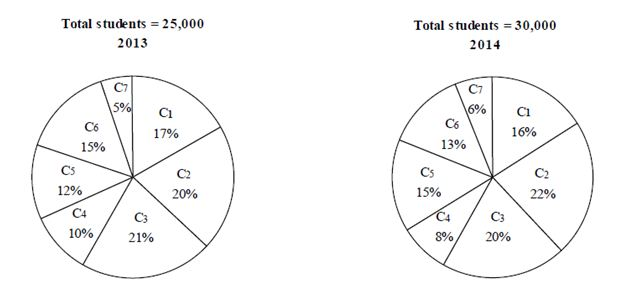# NIACL AO Prelims – Quantitative Aptitude Questions Day- 86

Dear Readers, Bank Exam Race for the Year 2019 is already started, To enrich your preparation here we have providing new series of Practice Questions on Quantitative Aptitude – Section. Candidates those who are preparing for NIACL AO Prelims 2019 Exams can practice these questions daily and make your preparation effective.

[WpProQuiz 5008]

Directions (1 – 5): What value should come in place of question mark (?) in the following questions?

1) √((36 ÷3)×(? ÷3)) = 7.2 ÷ 6 + 0.3

a) 0.5625

b) 0.3136

c) 0.2025

d) 0.4225

e) None of these

2) (16 × 10-7)2 ÷ (32 × 23 × 10-3) = 10?

a) -8

b) -6

c) -11

d) -12

e) None of these

3) 2197 ÷ 13 ÷ 2 × 18 + 48 % of 550 + 62 % of 1700 = x

a) 3765

b) 2839

c) 4721

d) 1967

e) None of these

4) (1.6)?-3 = (2.56)5 ÷ (4096 ÷ 1000)3 × (0.8 × 2)7

a) 8

b) 13

c) 6

d) 11

e) None of these

5) 75 % of (2/17) of (51/147) of (2/5) of 38465 =?

a) 345

b) 589

c) 251

d) 640

e) None of these

Directions (6 – 10): Study the following pie-charts carefully and answer the questions given below:

Number of students studying in different colleges in the year 2013 and 2014 from state ‘A’6) What was the percentage increase in the number of students in college C5 in the year 2014 while comparing with 2013?

a) 45%

b) 40%

c) 48%

d) 50%

e) 42%

7) In which of the following colleges was the per cent increase in the number of students, the minimum in 2014 while comparing with 2013?

a) C2

b) C6

c) C4

d) C7

e) C1

8) In which college there was decrease in the number of students from 2013 to 2014?

a) C6

b) C1

c) C4

d) C2

e) C3

9) In the year 2013, the number of students studying in college C1 and C2 together is approximately what percent of the number of students studying in these colleges together in 2014?

a) 78%

b) 75%

c) 72%

d) 81%

e) 88%

10) What was the ratio of the number of students studying in college C6 in year 2013 to that in 2014 in the same college?

a) 25: 26

b) 25: 27

c) 23: 26

d) 22: 27

e) 23: 25

Directions (1-5):

√((36 ÷3)×(x ÷3)) = 7.2 ÷ 6 + 0.3

√(4x) = 1.2+0.3

2√x = 1.5

√x = 1.5/2 = 0.75

X = 0.5625

(16*16*10-14)  ÷ (32 × 23 × 10-3) = 10x

10-14+3 = 10x

X = -11

2197 ÷ 13 ÷ 2 × 18 + 48 % of 550 + 62 % of 1700 = x

1521 + (48/100)*550 + (62/100)*1700 = x

X = 1521 + 264 + 1054

X = 2839

(1.6)x-3 = (1.6)10 ÷ (4.096)3 × (1.6)7

(1.6)x-3 = (1.6)10 ÷ (1.6)9 × (1.6)7

(1.6)x-3 = 1.610-9+7

(1.6)x-3 = 1.68

x-3 = 8

x = 11

(75/100)* (2/17) * (51/147) * (2/5) * 38465 = x

X = 471

Directions (6-10):

Number of students in college C5

In 2014 = 15/100 * 30000 = 4500

In 2013, 12/100 * 25000 = 3000

Required % Increase = (4500 – 3000)/3000 * 100 = 50%

For 2013:

C1 —- 17 × 250 = 4250

C2 —- 20 × 250 = 5000

C3 —- 21 × 250 = 5250

C4 —- 10 × 250 = 2500

C5 —- 12 × 250 = 3000

C6 —- 15 × 250 = 3750

C7 —- 5 × 250 = 1250

For 2014:

C1 —- 16 * 300 = 4800

C2 —- 22 * 300 = 6600

C3 —- 20 * 300 = 6000

C4 —- 8 * 300 = 2400

C5 —- 15 * 300 = 4500

C6 —- 13 * 300 = 3900

C7 —- 6 * 300 = 1800

Percentage Increase

C1 = (4800 – 4250)/4250 * 100 = 12.94%

C2 = (6600 – 5000)/5000 * 100 = 32 %

C3 = (6000 – 5250)/5250 * 100 = 14.28%

C4 = (2500 – 2400)/2500 * 100 = 4 % Decrease

C5 = (4500 – 3000)/3000 * 100 = 50%

C6 = (3900 – 3750)/3750 * 100 = 4%

C7 = (1800 – 1250)/1250 * 100 = 44 %

Hence, minimum increase is in the college C6

For 2013:

C1 —- 17 × 250 = 4250

C2 —- 20 × 250 = 5000

C3 —- 21 × 250 = 5250

C4 —- 10 × 250 = 2500

C5 —- 12 × 250 = 3000

C6 —- 15 × 250 = 3750

C7 —- 5 × 250 = 1250

For 2014:

C1 —- 16 * 300 = 4800

C2 —- 22 * 300 = 6600

C3 —- 20 * 300 = 6000

C4 —- 8 * 300 = 2400

C5 —- 15 * 300 = 4500

C6 —- 13 * 300 = 3900

C7 —- 6 * 300 = 1800

Percentage Increase

C1 = (4800 – 4250)/4250 * 100 = 12.94%

C2 = (6600 – 5000)/5000 * 100 = 32 %

C3 = (6000 – 5250)/5250 * 100 = 14.28%

C4 = (2500 – 2400)/2500 * 100 = 4 % Decrease

C5 = (4500 – 3000)/3000 * 100 = 50%

C6 = (3900 – 3750)/3750 * 100 = 4%

C7 = (1800 – 1250)/1250 * 100 = 44 %

Hence, decrease is in the college C4.